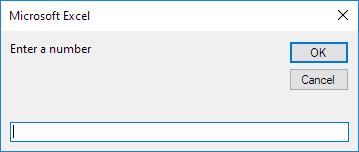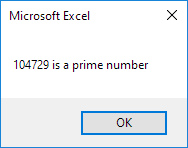# Prime Number Checker

Below we will look at a program in Excel VBA that checks whether a number is a prime number or not.

Before you start: in mathematics, a prime number is a number that has exactly two distinct number divisors: 1 and itself. The smallest twenty-five prime numbers are: 2, 3, 5, 7, 11, 13, 17, 19, 23, 29, 31, 37, 41, 43, 47, 53, 59, 61, 67, 71, 73, 79, 83, 89 and 97. For example, 8 has 1, 2, 4 and 8 as divisors and is not a prime number.

Situation:1. First, we declare three variables. One Integer variable we call divisors, one Long variable we call number and one Long variable we call i. We use Long variables here because Long variables have larger capacity than Integer variables.

Dim divisors As Integer, number As Long, i As Long

2. We initialize two variables. We initialize the variable divisors with value 0. We use the InputBox function to get a number from the user.

divisors = 0
number = InputBox("Enter a number")After the user has entered a number, we want to check whether this number is a prime number or not. Remember, a prime number has exactly two distinct number divisors: 1 and itself.

3. We start a For Next loop.

For i = 1 To number

4. Now comes the most important part of the program. To calculate the number of divisors of a number, we use the Mod operator. The Mod operator gives the remainder of a division. For example, 7 mod 2 = 1 because 7 divided by 2 equals 3 with a remainder of 1. Only if 'number mod i' = 0, i is a divisor of number. In this case we want to increment the variable divisors by 1. The macro below does the trick.

If number Mod i = 0 Then
divisors = divisors + 1
End If

Excel VBA checks this for i = 1, i = 2, i = 3, i = 4 until i = number. Note that i = 1 and i = number always are divisors of number. Only if these numbers are the only divisors of number, the number is a prime number.

5. Don't forget to close the loop.

Next i

6. If divisors equals 2, we display a msgbox saying that the entered number is a prime number. If the number of divisors is higher than 2, we display a msgbox saying that the entered number is not a prime number.

If divisors = 2 Then
MsgBox number & " is a prime number"
Else
MsgBox number & " is not a prime number"
End If

7. Test the program.

Result for 104729:Go to Next Chapter: Loop